#### Compliant motions

It will be seen shortly that the possibility of executing compliant motions is crucial for reducing uncertainty in the robot position. Let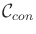denote the obstacle boundary,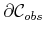(also,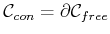). A model of robot motion while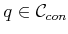needs to be formulated. In general, this is complicated by friction. A simple Coulomb friction model is assumed here; see  for more details on modeling friction in the context of manipulation planning. Suppose that the net force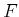is applied by a robot at some. The force could be maintained by using the generalized damper model of robot control .

The resulting motion is characterized using a friction cone, as shown in Figure 12.44a. A basic principle of Newtonian mechanics is that the obstacle applies a reaction force (it may be helpful to look ahead to Section 13.3, which introduces mechanics). Ifpoints into the surface and is normal to it, then the reaction force provided by the obstacle will cancel, and there will be no motion. Ifis not perpendicular to the surface, then sliding may occur. At one extreme,may be parallel to the surface. In this case, it must slide along the boundary. In general,can be decomposed into parallel and perpendicular components. If the parallel component is too small relative to the perpendicular component, then the robot becomes stuck. The friction cone shown in Figure 12.44a indicates precisely the conditions under which motion occurs. The parameter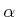captures the amount of friction (more friction leads to larger). Figure 12.44b indicates the behaviors that occur for various directions of. The diagram is obtained by inverting the friction cone. Ifpoints into the bottom region, then sticking occurs, which means that the robot cannot move. Ifpoints away from the obstacle boundary, then contact is broken (this is reasonable, unless the boundary is sticky). For the remaining two cases, the robot slides along the boundary.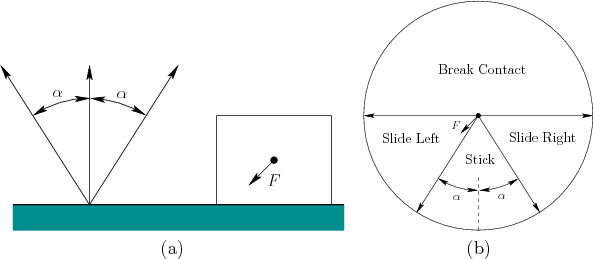Steven M LaValle 2020-08-14